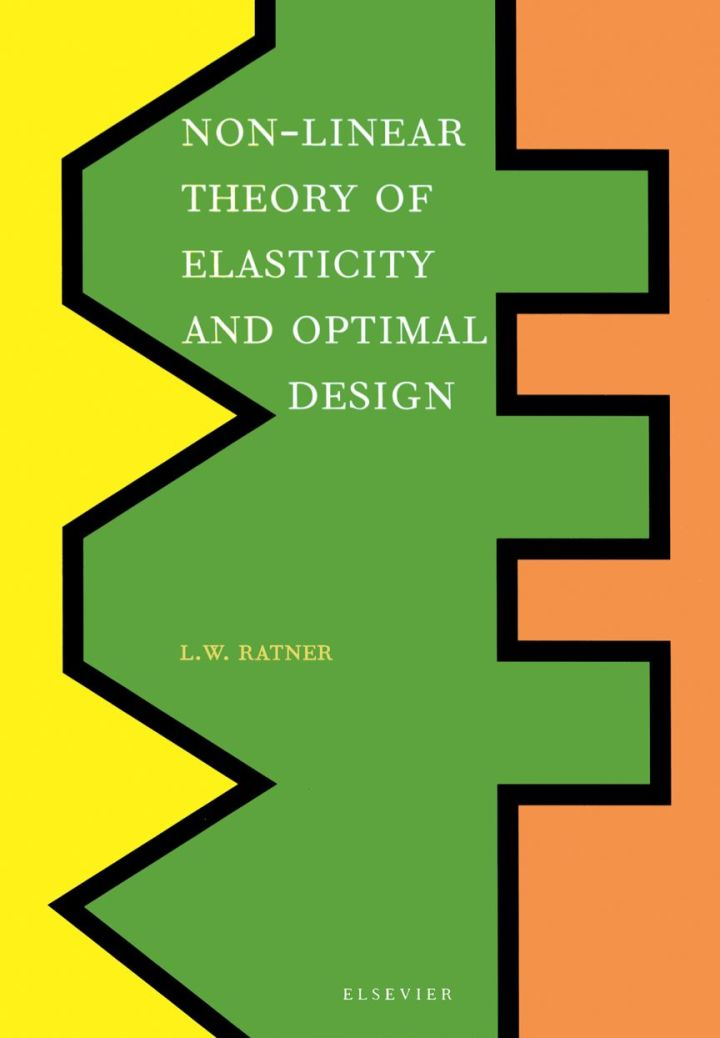# Non-Linear Theory of Elasticity and Optimal Design

## Non-Linear Theory of Elasticity and Optimal Design

By Ratner, L.W.

### Rent or Buy eTextbook

Expires on Apr 17th, 2022
\$185

Publisher List Price: \$189.99
Savings: \$4.99

In order to select an optimal structure among possible similar structures, one needs to compare the elastic behavior of the structures. A new criterion that describes elastic behavior is the rate of change of deformation. Using this criterion, the safe dimensions of a structure that are required by the stress distributed in a structure can be calculated. The new non-linear theory of elasticity allows one to determine the actual individual limit of elasticity/failure of a structure using a simple non-destructive method of measurement of deformation on the model of a structure while presently it can be done only with a destructive test for each structure. For building and explaining the theory, a new logical structure was introduced as the basis of the theory. One of the important physical implications of this logic is that it describes mathematically the universal domain of the possible stable physical relations.

Subject: Physical & Earth Sciences -> Physics -> GeneralPublisher: Elsevier S & T 11/2003
Imprint: Elsevier Science
Language: English
Length: 279 pages

ISBN 10: 0444514279
ISBN 13: 9780444514271
Print ISBN: 9780444514271

Live Chats